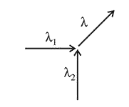# Two particles move at right angle to each other.

Question:

Two particles move at right angle to each other. Their de Broglie wavelengths are $\lambda_{1}$ and $\lambda_{2}$ respectively. The particles suffer perfectly inelastic collision. The de Broglie wavelength $\lambda$, of the final particle, is given by:

1. (1) $\frac{1}{\lambda^{2}}=\frac{1}{\lambda_{1}^{2}}+\frac{1}{\lambda_{2}^{2}}$

2. (2) $\lambda=\sqrt{\lambda_{1} \lambda_{2}}$

3. (3) $\lambda=\frac{\lambda_{2}+\lambda_{2}}{2}$

4. (4) $\frac{2}{\lambda}=\frac{1}{\lambda_{1}}+\frac{1}{\lambda_{2}}$

Correct Option: 1

Solution:

(1) From the de-Broglie relation,

$p_{1}=\frac{h}{\lambda_{1}}$

$p_{2}=\frac{h}{\lambda_{2}}$Momentum of the final particle $\left(\mathrm{p}_{\mathrm{f}}\right)$ is given by

$\therefore \quad p_{f}=\sqrt{p_{1}^{2}+p_{2}^{2}}$

$\Rightarrow \frac{h}{\lambda}=\sqrt{\frac{h^{2}}{\lambda_{1}^{2}}+\frac{h^{2}}{\lambda_{2}^{2}}}$

$\Rightarrow \frac{1}{\lambda^{2}}=\frac{1}{\lambda_{1}^{2}}+\frac{1}{\lambda_{2}^{2}}$